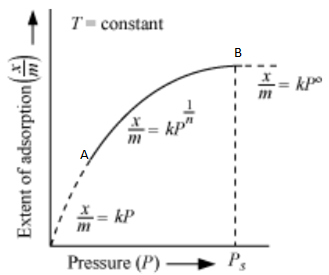# NEET Chemistry Surface Chemistry Questions Solved

BOARD

What is an adsorption isotherm? Describe Freundlich adsorption isotherm.

Adsorption isotherm: A graph between the amount of the gas adsorbed

per gram of the adsorbent (x/m) and the equlibrium pressure of the adsorbate

at constant temperature is called the adsorption isotherm.

The extent of adsorption is usually expressed as x/m,Variation of x/m with increase in pressure at constant temperature

(Freundlich adsorption isotherm)

A relationship between the amount adsorbed (x/m) and the equilibrium

pressure (P) can be obtained as follows:

At low values of P, the graph is nearly straight and sloping.

At high pressure, x/m becomes independent of the values of P.

In this range of pressure ,

In the intermediate range of pressure, x/m will depend on P raised to

powers between 1 and 0 i.e. fraction. For a small range of pressure values,

we can write:

where

[n=Positive integer

k=constant depends upon the nature of

adsorbate and adsorbent at a particular temperature.

The factor 1/n has values between 0 and1.]

This relationship was given by Freundlich and is known as Freundlich adsorption

isotherm.

Difficulty Level:

• 100%
• 0%